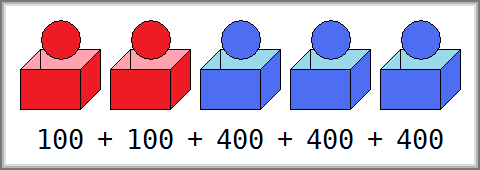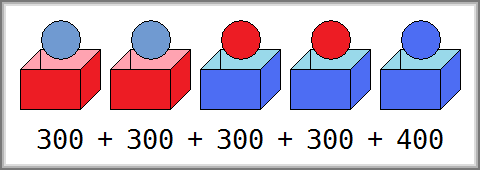### Problem Statement

You are playing a game where you have numRed red boxes, numBlue blue boxes, numRed red balls, and numBlue blue balls.

You must place a single ball into each box. Each box is then scored as follows:
• If the box is red and it contains a red ball, you get onlyRed points.
• If the box is blue and it contains a blue ball, you get onlyBlue points.
• In all other cases, you get bothColors points.
Your total score is the sum of the scores of all the boxes.

Return the maximum possible total score you can get.

### Definition

 Class: ColorfulBoxesAndBalls Method: getMaximum Parameters: int, int, int, int, int Returns: int Method signature: int getMaximum(int numRed, int numBlue, int onlyRed, int onlyBlue, int bothColors) (be sure your method is public)

### Constraints

-numRed and numBlue will each be between 1 and 100, inclusive.
-onlyRed, onlyBlue, and bothColors will each be between -1000 and 1000, inclusive.

### Examples

0)

 `2` `3` `100` `400` `200`
`Returns: 1400`In this example, you should put two red balls into red boxes, and three blue balls into blue boxes. Then you can get 100 * 2 + 400 * 3 = 1400 points in total.
1)

 `2` `3` `100` `400` `300`
`Returns: 1600`bothColors is a larger value here than it was in the previous example. You should put two blue balls into red boxes, and two red balls and one blue ball into blue boxes. Then you can get 300 * 4 + 400 * 1 = 1600 points.
2)

 `5` `5` `464` `464` `464`
`Returns: 4640`
 No matter how you place the balls, your score will always be the same.
3)

 `1` `4` `20` `-30` `-10`
`Returns: -100`
 The maximum total score may be less than zero.
4)

 `9` `1` `-1` `-10` `4`
`Returns: 0`

#### Problem url:

http://www.topcoder.com/stat?c=problem_statement&pm=10743

#### Problem stats url:

http://www.topcoder.com/tc?module=ProblemDetail&rd=14149&pm=10743

lyrically

#### Testers:

PabloGilberto , ivan_metelsky , it4.kp

#### Problem categories:

Simple Math, Simple Search, Iteration